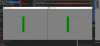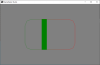# Legacy GMGM drawing acting in unexplainable ways

#### Tthecreator

Hi there!

I was working on my UI asset and came across a weird bug I just cannot wrap my head around.

I was making a function called draw_square_rounded_colour, which is a combination of draw_rectangle_colour and draw_roundrect. (draw_roundrect_colour only has 2 colors instead of 4).
I finished the function but there is a strange bug that only occurs at odd-numbered screen sizes. A few of the lines in my primitive seem to be two wide instead of one with 50% alpha.
The problem only occurs when I draw on a surface. My surface is always the same as my window size. I've disabled all my shaders and blend mode code. I'm also rounding all the x and y coordinates I put into my primitives. Everything is drawn in the GUI event, so it can't be any application_surface stuff.

Another very strange thing is that whenever I make a screenshot of my entire desktop I get a different result from just making a screenshot of a single window.This is what it should normally look like:It would be great is someone could help me explain and fix this behavior. I'm hoping someone has seen this before and can tell me about it.

Anyways, here is the script that I'm using to draw things:
Code:
``````///draw_square_rounded_colour(x1,y1,x2,y2,color1,color2,color3,color4,alpha,xstretch,ystretch,advancedBlending,outline)
/*
Does the same as game maker's draw_rectangle_colour, but this one is compatible with the uiz shaders.
Always try to use this function instead of draw_rectangle_colour if you are making an uiZ object.

In addition to draw_square_colour this has the following two extra arguments:
xstretch: (in px) the distance on the x-axis between the (imaginary) 90 degree corner and the point at which the curve stops.
ystretch: (in px) the distance on the y-axis between the (imaginary) 90 degree corner and the point at which the curve stops.
advancedBlending: if true a lower performance but better looking blending method is used. If higher a worse looking but slightly faster method is used.
*/
//draw_sprite_ext(spr_square,0,argument0,argument1,argument2-argument0,argument3-argument1,0,argument4,argument5)
draw_set_color(c_white)
var argument_arr = array_create(argument_count);
for (var i = 0; i < argument_count; i++) {
argument_arr[i] = argument[i];
}
if (live_call_ext(argument_arr)) return live_result;

//sdbm(argument0,argument1,argument2,argument3);

var width = argument2 - argument0;
var height = argument3 - argument1;
var xstretch = min(width/2,argument9)
var ystretch = min(height/2,argument10)
var precision = ceil(max(xstretch,ystretch)/7);
precision=2;

//if width > xstretch and height > ystretch then{ drawUsingAdvancedBlending = true; }//if the rounded square is big enough, the colors will start to look incorrect (a vague cross is visible). To counter this, advanced color blending is enabled. This will give a small performance hit (which is why it isn' t enabled by default)
if argument12 then{
draw_primitive_begin_texture(pr_linestrip,sprite_get_texture(spr_square,0))
}else{
draw_primitive_begin_texture(pr_trianglefan,sprite_get_texture(spr_square,0))
var middle_blend_r = (colour_get_red(argument4)+colour_get_red(argument5)+colour_get_red(argument6)+colour_get_red(argument7))/4
var middle_blend_g = (colour_get_green(argument4)+colour_get_green(argument5)+colour_get_green(argument6)+colour_get_green(argument7))/4
var middle_blend_b = (colour_get_blue(argument4)+colour_get_blue(argument5)+colour_get_blue(argument6)+colour_get_blue(argument7))/4
draw_vertex_texture_colour((argument0+argument2)/2,(argument1+argument3)/2,0,0,make_color_rgb(middle_blend_r,middle_blend_g,middle_blend_b),argument8)//middle
}

if drawUsingAdvancedBlending = false or true then{
//draw_vertex_texture_colour(argument0+xstretch,argument1,0,0,argument4,argument8)//top left (right side of curve)
for(var i=-precision;i<=0;++i){//curve top right
var x_offset = dcos(i/precision*90)*xstretch;
var y_offset = dsin(i/precision*90)*ystretch;
var x_pos = round(argument2-xstretch+x_offset);
var y_pos = round(argument1+ystretch+y_offset);
draw_vertex_texture_colour(x_pos,y_pos,1,0,argument5,argument8)
}

for(var i=0;i<=precision;++i){//curve bottom right
var x_offset = dcos(i/precision*90)*xstretch;
var y_offset = dsin(i/precision*90)*ystretch;
var x_pos = round(argument2-xstretch+x_offset);
var y_pos = round(argument3-ystretch+y_offset);
draw_vertex_texture_colour(x_pos,y_pos,0.8,0.8,argument6,argument8)
}

for(var i=precision;i<=precision*2;++i){//curve bottom left
var x_offset = dcos(i/precision*90)*xstretch;
var y_offset = dsin(i/precision*90)*ystretch;
var x_pos = round(argument0+xstretch+x_offset);
var y_pos = round(argument3-ystretch+y_offset);
draw_vertex_texture_colour(x_pos,y_pos,0.2,0.8,argument7,argument8)
}

for(var i=precision*2;i<=precision*3;++i){//curve top left
var x_offset = dcos(i/precision*90)*xstretch;
var y_offset = dsin(i/precision*90)*ystretch;
var x_pos = round(argument0+xstretch+x_offset);
var y_pos = round(argument1+ystretch+y_offset);
draw_vertex_texture_colour(x_pos,y_pos,0.2,0.2,argument4,argument8)
}
draw_vertex_texture_colour(round(argument2-xstretch),round(argument1),0.8,0.2,argument5,argument8)//top right (left side of curve)
}else{
//draw_vertex_texture_colour(argument0+xstretch,argument1,0,0,argument4,argument8)//top left (right side of curve)
for(var i=-precision;i<=0;++i){//curve top right
var x_offset = dcos(i/precision*90)*xstretch;
var y_offset = dsin(i/precision*90)*ystretch;
var x_pos = argument2-xstretch+x_offset;
var y_pos = argument1+ystretch+y_offset;
var col = draw_square_rounded_colour_interpolate((x_pos-argument0)/width,(y_pos-argument1)/height,argument4,argument5,argument6,argument7);
draw_vertex_texture_colour(x_pos,y_pos,1,0,col,argument8)
}

for(var i=0;i<=precision;++i){//curve bottom right
var x_offset = dcos(i/precision*90)*xstretch;
var y_offset = dsin(i/precision*90)*ystretch;
var x_pos = argument2-xstretch+x_offset;
var y_pos = argument3-ystretch+y_offset;
var col = draw_square_rounded_colour_interpolate((x_pos-argument0)/width,(y_pos-argument1)/height,argument4,argument5,argument6,argument7);
draw_vertex_texture_colour(x_pos,y_pos,1,1,col,argument8)
}

for(var i=precision;i<=precision*2;++i){//curve bottom left
var x_offset = dcos(i/precision*90)*xstretch;
var y_offset = dsin(i/precision*90)*ystretch;
var x_pos = argument0+xstretch+x_offset;
var y_pos = argument3-ystretch+y_offset;
var col = draw_square_rounded_colour_interpolate((x_pos-argument0)/width,(y_pos-argument1)/height,argument4,argument5,argument6,argument7);
draw_vertex_texture_colour(x_pos,y_pos,0,1,col,argument8)
}

for(var i=precision*2;i<=precision*3;++i){//curve top left
var x_offset = dcos(i/precision*90)*xstretch;
var y_offset = dsin(i/precision*90)*ystretch;
var x_pos = argument0+xstretch+x_offset;
var y_pos = argument1+ystretch+y_offset;
var col = draw_square_rounded_colour_interpolate((x_pos-argument0)/width,(y_pos-argument1)/height,argument4,argument5,argument6,argument7);
draw_vertex_texture_colour(x_pos,y_pos,0,0,col,argument8)
}
var col = draw_square_rounded_colour_interpolate((width-xstretch)/width,0,argument4,argument5,argument6,argument7);
draw_vertex_texture_colour(argument2-xstretch,argument1,1,0,col,argument8)//top right (left side of curve)
}
draw_primitive_end()``````

I am using GMS1.4.9999 with GMLive.

#### Juju

##### Member
Line drawing is suuuuper inaccurate on GPUs and generally shouldn't be relied upon. Does this bug still occur when using filled triangles?

#### Tthecreator

@Juju thanks for the answer. I've been able to solve the first bug by just making sure the window is always evenly sized. Also the reason the print screens acted differently was because I had some debug code activate when I was pressing cntrl.

I'm drawing a blue sprite on my screen and I'm drawing my roundrect on top of that (roundrect goes from 256;192 to 768;576).